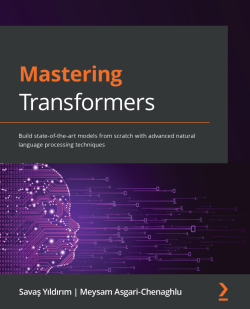# Mastering Transformers5 (3 reviews total)
By Savaş Yıldırım , Meysam Asgari-Chenaghlu
• \$41.99 Print
• \$33.99 eBook
• \$12.99 eBook + Subscription
What do you get with a Packt Subscription?

• Constantly updated with 100+ new titles each month
• Breadth and depth in over 1,000+ technologies

Transformer-based language models have dominated natural language processing (NLP) studies and have now become a new paradigm. With this book, you'll learn how to build various transformer-based NLP applications using the Python Transformers library.

The book gives you an introduction to Transformers by showing you how to write your first hello-world program. You'll then learn how a tokenizer works and how to train your own tokenizer. As you advance, you'll explore the architecture of autoencoding models, such as BERT, and autoregressive models, such as GPT. You'll see how to train and fine-tune models for a variety of natural language understanding (NLU) and natural language generation (NLG) problems, including text classification, token classification, and text representation. This book also helps you to learn efficient models for challenging problems, such as long-context NLP tasks with limited computational capacity. You'll also work with multilingual and cross-lingual problems, optimize models by monitoring their performance, and discover how to deconstruct these models for interpretability and explainability. Finally, you'll be able to deploy your transformer models in a production environment.

By the end of this NLP book, you'll have learned how to use Transformers to solve advanced NLP problems using advanced models.

Publication date:
September 2021
Publisher
Packt
Pages
374
ISBN
9781801077651

# Chapter 1: From Bag-of-Words to the Transformer

In this chapter, we will discuss what has changed in Natural Language Processing (NLP) over two decades. We experienced different paradigms and finally entered the era of Transformer architectures. All the paradigms help us to gain a better representation of words and documents for problem-solving. Distributional semantics describes the meaning of a word or a document with vectorial representation, looking at distributional evidence in a collection of articles. Vectors are used to solve many problems in both supervised and unsupervised pipelines. For language-generation problems, n-gram language models have been leveraged as a traditional approach for years. However, these traditional approaches have many weaknesses that we will discuss throughout the chapter.

We will further discuss classical Deep Learning (DL) architectures such as Recurrent Neural Networks (RNNs), Feed-Forward Neural Networks (FFNNs), and Convolutional Neural Networks (CNNs). These have improved the performance of the problems in the field and have overcome the limitation of traditional approaches. However, these models have had their own problems too. Recently, Transformer models have gained immense interest because of their effectiveness in all NLP tasks, from text classification to text generation. However, the main success has been that Transformers effectively improve the performance of multilingual and multi-task NLP problems, as well as monolingual and single tasks. These contributions have made Transfer Learning (TL) more possible in NLP, which aims to make models reusable for different tasks or different languages.

Starting with the attention mechanism, we will briefly discuss the Transformer architecture and the differences between previous NLP models. In parallel with theoretical discussions, we will show practical examples with the popular NLP framework. For the sake of simplicity, we will choose introductory code examples that are as short as possible.

In this chapter, we will cover the following topics:

• Evolution of NLP toward Transformers
• Understanding distributional semantics
• Leveraging DL
• Overview of the Transformer architecture
• Using TL with Transformers

# Technical requirements

We will be using Jupyter Notebook to run our coding exercises that require `python >=3.6.0`, along with the following packages that need to be installed with the `pip install` command:

• `sklearn`
• `nltk==3.5.0`
• `gensim==3.8.3`
• `fasttext`
• `keras>=2.3.0`
• `Transformers >=4.00`

All notebooks with coding exercises are available at the following GitHub link: https://github.com/PacktPublishing/Advanced-Natural-Language-Processing-with-Transformers/tree/main/CH01.

Check out the following link to see Code in Action Video: https://bit.ly/2UFPuVd

# Evolution of NLP toward Transformers

We have seen profound changes in NLP over the last 20 years. During this period, we experienced different paradigms and finally entered a new era dominated mostly by magical Transformer architecture. This architecture did not come out of nowhere. Starting with the help of various neural-based NLP approaches, it gradually evolved to an attention-based encoder-decoder type architecture and still keeps evolving. The architecture and its variants have been successful thanks to the following developments in the last decade:

• Contextual word embeddings
• Better subword tokenization algorithms for handling unseen words or rare words
• Injecting additional memory tokens into sentences, such as `Paragraph ID` in `Doc2vec` or a Classification (CLS) token in Bidirectional Encoder Representations from Transformers (BERT)
• Attention mechanisms, which overcome the problem of forcing input sentences to encode all information into one context vector
• Positional encoding to case word order
• Parallelizable architectures that make for faster training and fine-tuning
• Model compression (distillation, quantization, and so on)

For many years, we used traditional NLP approaches such as n-gram language models, TF-IDF-based information retrieval models, and one-hot encoded document-term matrices. All these approaches have contributed a lot to the solution of many NLP problems such as sequence classification, language generation, language understanding, and so forth. On the other hand, these traditional NLP methods have their own weaknesses—for instance, falling short in solving the problems of sparsity, unseen words representation, tracking long-term dependencies, and others. In order to cope with these weaknesses, we developed DL-based approaches such as the following:

• RNNs
• CNNs
• FFNNs
• Several variants of RNNs, CNNs, and FFNNs

In 2013, as a two-layer FFNN word-encoder model, `Word2vec`, sorted out the dimensionality problem by producing short and dense representations of the words, called word embeddings. This early model managed to produce fast and efficient static word embeddings. It transformed unsupervised textual data into supervised data (self-supervised learning) by either predicting the target word using context or predicting neighbor words based on a sliding window. GloVe, another widely used and popular model, argued that count-based models can be better than neural models. It leverages both global and local statistics of a corpus to learn embeddings based on word-word co-occurrence statistics. It performed well on some syntactic and semantic tasks, as shown in the following screenshot. The screenshot tells us that the embeddings offsets between the terms help to apply vector-oriented reasoning. We can learn the generalization of gender relations, which is a semantic relation from the offset between man and woman (man-> woman). Then, we can arithmetically estimate the vector of actress by adding the vector of the term actor and the offset calculated before. Likewise, we can learn syntactic relations such as word plural forms. For instance, if the vectors of Actor, Actors, and Actress are given, we can estimate the vector of Actresses: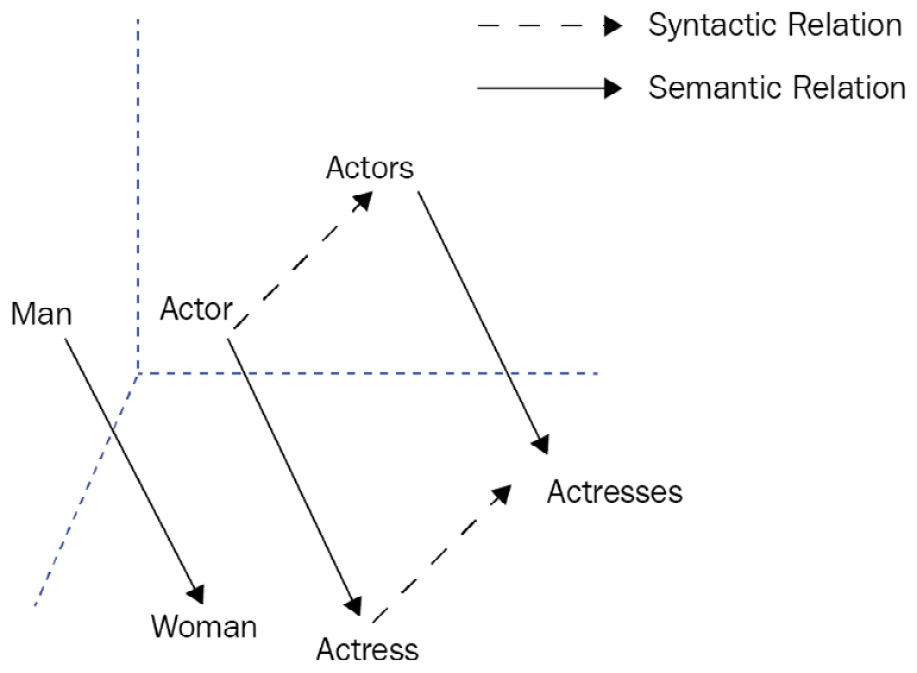Figure 1.1 – Word embeddings offset for relation extraction

The recurrent and convolutional architectures such as RNN, Long Short-Term Memory (LSTM), and CNN started to be used as encoders and decoders in sequence-to-sequence (seq2seq) problems. The main challenge with these early models was polysemous words. The senses of the words are ignored since a single fixed representation is assigned to each word, which is especially a severe problem for polysemous words and sentence semantics.

The further pioneer neural network models such as Universal Language Model Fine-tuning (ULMFit) and Embeddings from Language Models (ELMo) managed to encode the sentence-level information and finally alleviate polysemy problems, unlike with static word embeddings. These two important approaches were based on LSTM networks. They also introduced the concept of pre-training and fine-tuning. They help us to apply TL, employing the pre-trained models trained on a general task with huge textual datasets. Then, we can easily perform fine-tuning by resuming training of the pre-trained network on a target task with supervision. The representations differ from traditional word embeddings such that each word representation is a function of the entire input sentence. The modern Transformer architecture took advantage of this idea.

In the meantime, the idea of an attention mechanism made a strong impression in the NLP field and achieved significant success, especially in seq2seq problems. Earlier methods would pass the last state (known as a context vector or thought vector) obtained from the entire input sequence to the output sequence without linking or elimination. The attention mechanism was able to build a more sophisticated model by linking the tokens determined from the input sequence to the particular tokens in the output sequence. For instance, suppose you have a keyword phrase `Government of Canada` in the input sentence for an English to Turkish translation task. In the output sentence, the `Kanada Hükümeti` token makes strong connections with the input phrase and establishes a weaker connection with the remaining words in the input, as illustrated in the following screenshot:Figure 1.2 – Sketchy visualization of an attention mechanism

So, this mechanism makes models more successful in seq2seq problems such as translation, question answering, and text summarization.

In 2017, the Transformer-based encoder-decoder model was proposed and found to be successful. The design is based on an FFNN by discarding RNN recurrency and using only attention mechanisms (Vaswani et al., All you need is attention, 2017). The Transformer-based models have so far overcome many difficulties that other approaches faced and have become a new paradigm. Throughout this book, you will be exploring and understanding how the Transformer-based models work.

# Understanding distributional semantics

Distributional semantics describes the meaning of a word with a vectorial representation, preferably looking at its distributional evidence rather than looking at its predefined dictionary definitions. The theory suggests that words co-occurring together in a similar environment tend to share similar meanings. This was first formulated by the scholar Harris (Distributional Structure Word, 1954). For example, similar words such as dog and cat mostly co-occur in the same context. One of the advantages of a distributional approach is to help the researchers to understand and monitor the semantic evolution of words across time and domains, also known as the lexical semantic change problem.

Traditional approaches have applied Bag-of-Words (BoW) and n-gram language models to build the representation of words and sentences for many years. In a BoW approach, words and documents are represented with a one-hot encoding as a sparse way of representation, also known as the Vector Space Model (VSM).

Text classification, word similarity, semantic relation extraction, word-sense disambiguation, and many other NLP problems have been solved by these one-hot encoding techniques for years. On the other hand, n-gram language models assign probabilities to sequences of words so that we can either compute the probability that a sequence belongs to a corpus or generate a random sequence based on a given corpus.

## BoW implementation

A BoW is a representation technique for documents by counting the words in them. The main data structure of the technique is a document-term matrix. Let's see a simple implementation of BoW with Python. The following piece of code illustrates how to build a document-term matrix with the Python `sklearn` library for a toy corpus of three sentences:

```from sklearn.feature_extraction.text import TfidfVectorizer
import numpy as np
import pandas as pd
toy_corpus= ["the fat cat sat on the mat",
"the big cat slept",
"the dog chased a cat"]
vectorizer=TfidfVectorizer()
corpus_tfidf=vectorizer.fit_transform(toy_corpus)
print(f"The vocabulary size is \
{len(vectorizer.vocabulary_.keys())} ")
print(f"The document-term matrix shape is\
{corpus_tfidf.shape}")
df=pd.DataFrame(np.round(corpus_tfidf.toarray(),2))
df.columns=vectorizer.get_feature_names()```

The output of the code is a document-term matrix, as shown in the following screenshot. The size is (3 x 10), but in a realistic scenario the matrix size can grow to much larger numbers such as 10K x 10M: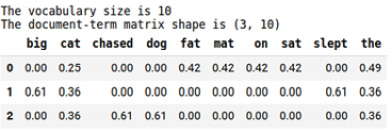Figure 1.3 – Document-term matrix

The table indicates a count-based mathematical matrix where the cell values are transformed by a Term Frequency-Inverse Document Frequency (TF-IDF) weighting schema. This approach does not care about the position of words. Since the word order strongly determines the meaning, ignoring it leads to a loss of meaning. This is a common problem in a BoW method, which is finally solved by a recursion mechanism in RNN and positional encoding in Transformers.

Each column in the matrix stands for the vector of a word in the vocabulary, and each row stands for the vector of a document. Semantic similarity metrics can be applied to compute the similarity or dissimilarity of the words as well as documents. Most of the time, we use bigrams such as `cat_sat` and `the_street` to enrich the document representation. For instance, as the parameter `ngram_range=(1,2)` is passed to `TfidfVectorizer`, it builds a vector space containing both unigrams (`big, cat, dog`) and bigrams (`big_cat`, `big_dog`). Thus, such models are also called bag-of-n-grams, which is a natural extension of BoW.

If a word is commonly used in each document, it can be considered to be high-frequency, such as and the. Conversely, some words hardly appear in documents, called low-frequency (or rare) words. As high-frequency and low-frequency words may prevent the model from working properly, TF-IDF, which is one of the most important and well-known weighting mechanisms, is used here as a solution.

Inverse Document Frequency (IDF) is a statistical weight to measure the importance of a word in a document—for example, while the word `the` has no discriminative power, `chased` can be highly informative and give clues about the subject of the text. This is because high-frequency words (stopwords, functional words) have little discriminating power in understanding the documents.

The discriminativeness of the terms also depends on the domain—for instance, a list of DL articles is most likely to have the word `network` in almost every document. IDF can scale down the weights of all terms by using their Document Frequency (DF), where the DF of a word is computed by the number of documents in which a term appears. Term Frequency (TF) is the raw count of a term (word) in a document.

Some of the advantages and disadvantages of a TF-IDF based BoW model are listed as follows: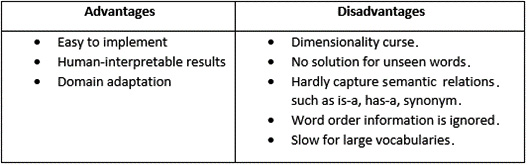## Overcoming the dimensionality problem

To overcome the dimensionality problem of the BoW model, Latent Semantic Analysis (LSA) is widely used for capturing semantics in a low-dimensional space. It is a linear method that captures pairwise correlations between terms. LSA-based probabilistic methods can be still considered as a single layer of hidden topic variables. However, current DL models include multiple hidden layers, with billions of parameters. In addition to that, Transformer-based models showed that they can discover latent representations much better than such traditional models.

For the Natural Language Understanding (NLU) tasks, the traditional pipeline starts with some preparation steps, such as tokenization, stemming, noun phrase detection, chunking, stop-word elimination, and much more. Afterward, a document-term matrix is constructed with any weighting schema, where TF-IDF is the most popular one. Finally, the matrix is served as a tabulated input for Machine Learning (ML) pipelines, sentiment analysis, document similarity, document clustering, or measuring the relevancy score between a query and a document. Likewise, terms are represented as a tabular matrix and can be input for a token classification problem where we can apply named-entity recognition, semantic relation extractions, and so on.

The classification phase includes a straightforward implementation of supervised ML algorithms such as Support Vector Machine (SVM), Random forest, logistic, naive bayes, and Multiple Learners (Boosting or Bagging). Practically, the implementation of such a pipeline can simply be coded as follows:

```from sklearn.pipeline import make_pipeline
from sklearn.svm import SVC
labels= [0,1,0]
clf = SVC()
clf.fit(df.to_numpy(), labels)```

As seen in the preceding code, we can apply fit operations easily thanks to the `sklearn` Application Programming Interface (API). In order to apply the learned model to train data, the following code is executed:

```clf.predict(df.to_numpy())
Output: array([0, 1, 0])```

Let's move on to the next section!

## Language modeling and generation

For language-generation problems, the traditional approaches are based on leveraging n-gram language models. This is also called a Markov process, which is a stochastic model in which each word (event) depends on a subset of previous words—unigram, bigram, or n-gram, outlined as follows:

• Unigram (all words are independent and no chain): This estimates the probability of word in a vocabulary simply computed by the frequency of it to the total word count.
• Bigram (First-order Markov process): This estimates the P (wordi| wordi-1).probability of wordi depending on wordi-1, which is simply computed by the ratio of P (wordi , wordi-1) to P (wordi-1).
• Ngram (N-order Markov process): This estimates P (wordi | word0, ..., wordi-1).

Let's give a simple language model implementation with the Natural Language Toolkit (NLTK) library. In the following implementation, we train a Maximum Likelihood Estimator (MLE) with order n=2. We can select any n-gram order such as n=1 for unigrams, n=2 for bigrams, n=3 for trigrams, and so forth:

```import nltk
from nltk.corpus import gutenberg
from nltk.lm import MLE
macbeth = gutenberg.sents('shakespeare-macbeth.txt')
lm=MLE(2)
lm.fit(model,vocab)
print(list(lm.vocab)[:10])
print(f"The number of words is {len(lm.vocab)}")```

The `nltk` package first downloads the `gutenberg` corpus, which includes some texts from the Project Gutenberg electronic text archive, hosted at https://www.gutenberg.org. It also downloads the `punkt` tokenizer tool for the punctuation process. This tokenizer divides a raw text into a list of sentences by using an unsupervised algorithm. The `nltk` package already includes a pre-trained English `punkt` tokenizer model for abbreviation words and collocations. It can be trained on a list of texts in any language before use. In the further chapters, we will discuss how to train different and more efficient tokenizers for Transformer models as well. The following code produces what the language model learned so far:

```print(f"The frequency of the term 'Macbeth' is {lm.counts['Macbeth']}")
print(f"The language model probability score of 'Macbeth' is {lm.score('Macbeth')}")
print(f"The number of times 'Macbeth' follows 'Enter' is {lm.counts[['Enter']]['Macbeth']} ")
print(f"P(Macbeth | Enter) is {lm.score('Macbeth', ['Enter'])}")
print(f"P(shaking | for) is {lm.score('shaking', ['for'])}")```

This is the output:

```The frequency of the term 'Macbeth' is 61
The language model probability score of 'Macbeth' is 0.00226
The number of times 'Macbeth' follows 'Enter' is 15
P(Macbeth | Enter) is 0.1875
P(shaking | for) is 0.0121```

The n-gram language model keeps n-gram counts and computes the conditional probability for sentence generation. `lm=MLE(2)` stands for MLE, which yields the maximum probable sentence from each token probability. The following code produces a random sentence of 10 words with the `<s>` starting condition given:

`lm.generate(10, text_seed=['<s>'], random_seed=42)`

The output is shown in the following snippet:

`['My', 'Bosome', 'franchis', "'", 's', 'of', 'time', ',', 'We', 'are']`

We can give a specific starting condition through the `text_seed` parameter, which makes the generation be conditioned on the preceding context. In our preceding example, the preceding context is `<s>`, which is a special token indicating the beginning of a sentence.

So far, we have discussed paradigms underlying traditional NLP models and provided very simple implementations with popular frameworks. We are now moving to the DL section to discuss how neural language models shaped the field of NLP and how neural models overcome the traditional model limitations.

# Leveraging DL

NLP is one of the areas where DL architectures have been widely and successfully used. For decades, we have witnessed successful architectures, especially in word and sentence representation. In this section, we will share the story of these different approaches with commonly used frameworks.

## Learning word embeddings

Neural network-based language models effectively solved feature representation and language modeling problems since it became possible to train more complex neural architecture on much larger datasets to build short and dense representations. In 2013, the Word2vec model, which is a popular word-embedding technique, used a simple and effective architecture to learn a high quality of continuous word representations. It outperformed other models for a variety of syntactic and semantic language tasks such as sentiment analysis, paraphrase detection, relation extraction, and so forth. The other key factor in the popularity of the model is its much lower computational complexity. It maximizes the probability of the current word given any surrounding context words, or vice versa.

The following piece of code illustrates how to train word vectors for the sentences of the play Macbeth:

```from gensim.models import Word2vec
model = Word2vec(sentences=macbeth, size=100, window= 4, min_count=10, workers=4, iter=10)```

The code trains the word embeddings with a vector size of 100 by a sliding 5-length context window. To visualize the words embeddings, we need to reduce the dimension to 3 by applying Principal Component Analysis (PCA) as shown in the following code snippet:

```import matplotlib.pyplot as plt
from sklearn.decomposition import PCA
import random
np.random.seed(42)
words=list([e for e in model.wv.vocab if len(e)>4])
random.shuffle(words)
words3d = PCA(n_components=3,random_state=42).fit_transform(model.wv[words[:100]])
def plotWords3D(vecs, words, title):
...
plotWords3D(words3d, words, "Visualizing Word2vec Word Embeddings using PCA")```

This is the output: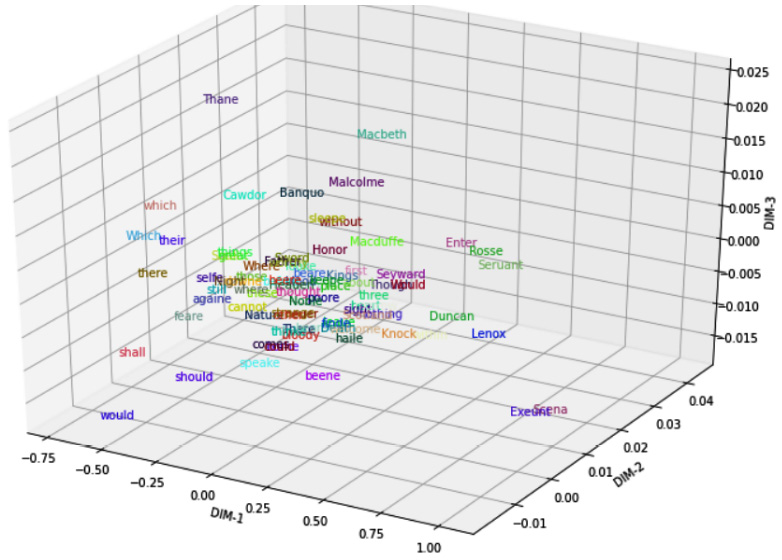Figure 1.4 – Visualizing word embeddings with PCA

As the plot shows, the main characters of Shakespeare's play—Macbeth, Malcolm, Banquo, Macduff, and others—are mapped close to each other. Likewise, auxiliary verbs shall, should, and would appear close to each other at the left-bottom of Figure 1.4. We can also capture an analogy such as man-woman= uncle-aunt by using an embedding offset. For more interesting visual examples on this topic, please check the following project: https://projector.tensorflow.org/.

The Word2vec-like models learn word embeddings by employing a prediction-based neural architecture. They employ gradient descent on some objective functions and nearby word predictions. While traditional approaches apply a count-based method, neural models design a prediction-based architecture for distributional semantics. Are count-based methods or prediction-based methods the best for distributional word representations? The GloVe approach addressed this problem and argued that these two approaches are not dramatically different. Jeffrey Penington et al. even supported the idea that the count-based methods could be more successful by capturing global statistics. They stated that GloVe outperformed other neural network language models on word analogy, word similarity, and Named Entity Recognition (NER) tasks.

These two paradigms, however, did not provide a helpful solution for unseen words and word-sense problems. They do not exploit subword information, and therefore cannot learn the embeddings of rare and unseen words.

FastText, another widely used model, proposed a new enriched approach using subword information, where each word is represented as a bag of character n-grams. The model sets a constant vector to each character n-gram and represents words as the sum of their sub-vectors, which is an idea that was first introduced by Hinrich Schütze (Word Space, 1993). The model can compute word representations even for unseen words and learn the internal structure of words such as suffixes/affixes, which is especially important with morphologically rich languages such as Finnish, Hungarian, Turkish, Mongolian, Korean, Japanese, Indonesian, and so forth. Currently, modern Transformer architectures use a variety of subword tokenization methods such as WordPiece, SentencePiece, or Byte-Pair Encoding (BPE).

## A brief overview of RNNs

RNN models can learn each token representation by rolling up the information of other tokens at an earlier timestep and learn sentence representation at the last timestep. This mechanism has been found beneficial in many ways, outlined as follows:

• Firstly, RNN can be redesigned in a one-to-many model for language generation or music generation.
• Secondly, many-to-one models can be used for text classification or sentiment analysis.
• And lastly, many-to-many models are used for NER problems. The second use of many-to-many models is to solve encoder-decoder problems such as machine translation, question answering, and text summarization.

As with other neural network models, RNN models take tokens produced by a tokenization algorithm that breaks down the entire raw text into atomic units also called tokens. Further, it associates the token units with numeric vectors—token embeddings—which are learned during the training. As an alternative, we can assign the embedded learning task to the well-known word-embedding algorithms such as Word2vec or FastText in advance.

Here is a simple example of an RNN architecture for the sentence `The cat is sad.`, where x0 is the vector embeddings of `the`, x1 is the vector embeddings of `cat`, and so forth. Figure 1.5 illustrates an RNN being unfolded into a full Deep Neural Network (DNN).

Unfolding means that we associate a layer to each word. For the `The cat is sad.` sequence, we take care of a sequence of five words. The hidden state in each layer acts as the memory of the network. It encodes information about what happened in all previous timesteps and in the current timestep. This is represented in the following diagram: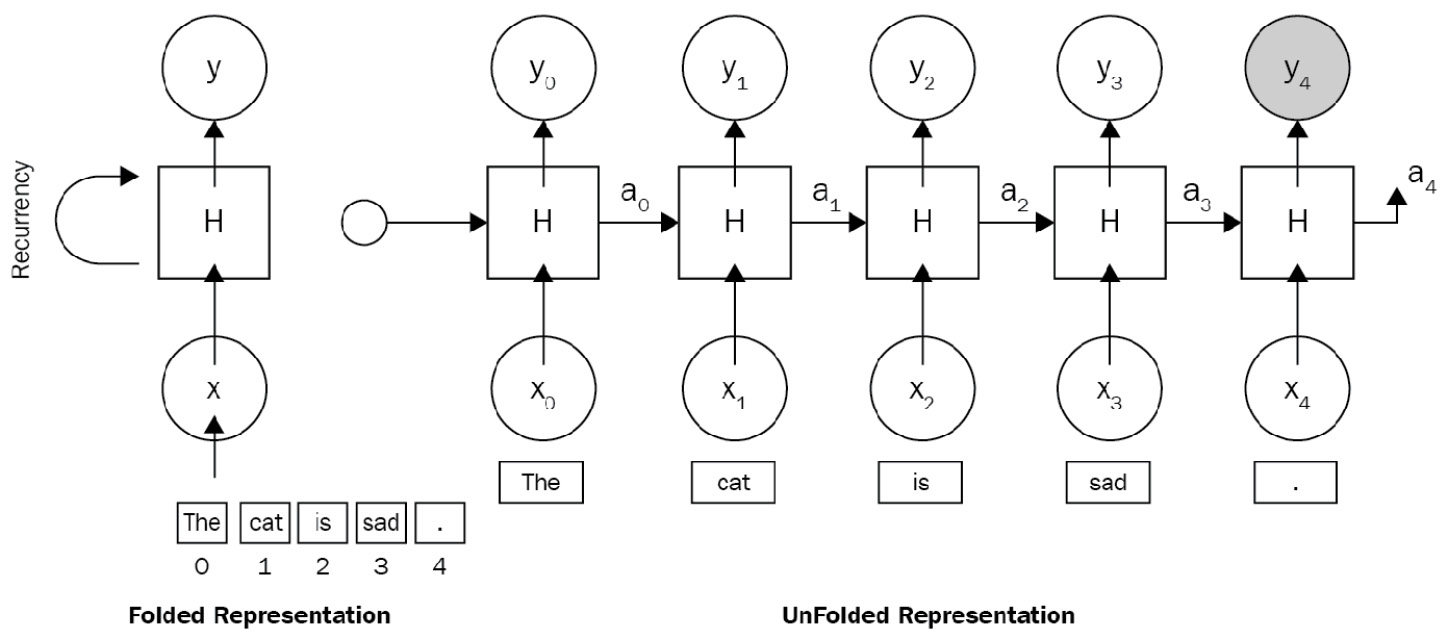Figure 1.5 – An RNN architecture

The following are some advantages of an RNN architecture:

• Variable-length input: The capacity to work on variable-length input, no matter the size of the sentence being input. We can feed the network with sentences of 3 or 300 words without changing the parameter.
• Caring about word order: It processes the sequence word by word in order, caring about the word position.
• Suitable for working in various modes (many-to-many, one-to-many): We can train a machine translation model or sentiment analysis using the same recurrency paradigm. Both architectures would be based on an RNN.

The disadvantages of an RNN architecture are listed here:

• Long-term dependency problem: When we process a very long document and try to link the terms that are far from each other, we need to care about and encode all irrelevant other terms between these terms.
• Prone to exploding or vanishing gradient problems: When working on long documents, updating the weights of the very first words is a big deal, which makes a model untrainable due to a vanishing gradient problem.
• Hard to apply parallelizable training: Parallelization breaks the main problem down into a smaller problem and executes the solutions at the same time, but RNN follows a classic sequential approach. Each layer strongly depends on previous layers, which makes parallelization impossible.
• The computation is slow as the sequence is long: An RNN could be very efficient for short text problems. It processes longer documents very slowly, besides the long-term dependency problem.

Although an RNN can theoretically attend the information at many timesteps before, in the real world, problems such as long documents and long-term dependencies are impossible to discover. Long sequences are represented within many deep layers. These problems have been addressed by many studies, some of which are outlined here:

• Hochreiter and Schmidhuber. Long Short-term Memory. 1997.
• Bengio et al. Learning long-term dependencies with gradient descent is difficult. 1993.
• K. Cho et al. Learning phrase representations using RNN encoder-decoder for statistical machine translation. 2014.

## LSTMs and gated recurrent units

LSTM (Schmidhuber, 1997) and Gated Recurrent Units (GRUs) (Cho, 2014) are new variants of RNNs, have solved long-term dependency problems, and have attracted great attention. LSTMs were particularly developed to cope with the long-term dependency problem. The advantage of an LSTM model is that it uses the additional cell state, which is a horizontal sequence line on the top of the LSTM unit. This cell state is controlled by special purpose gates for forget, insert, or update operations. The complex unit of an LSTM architecture is depicted in the following diagram: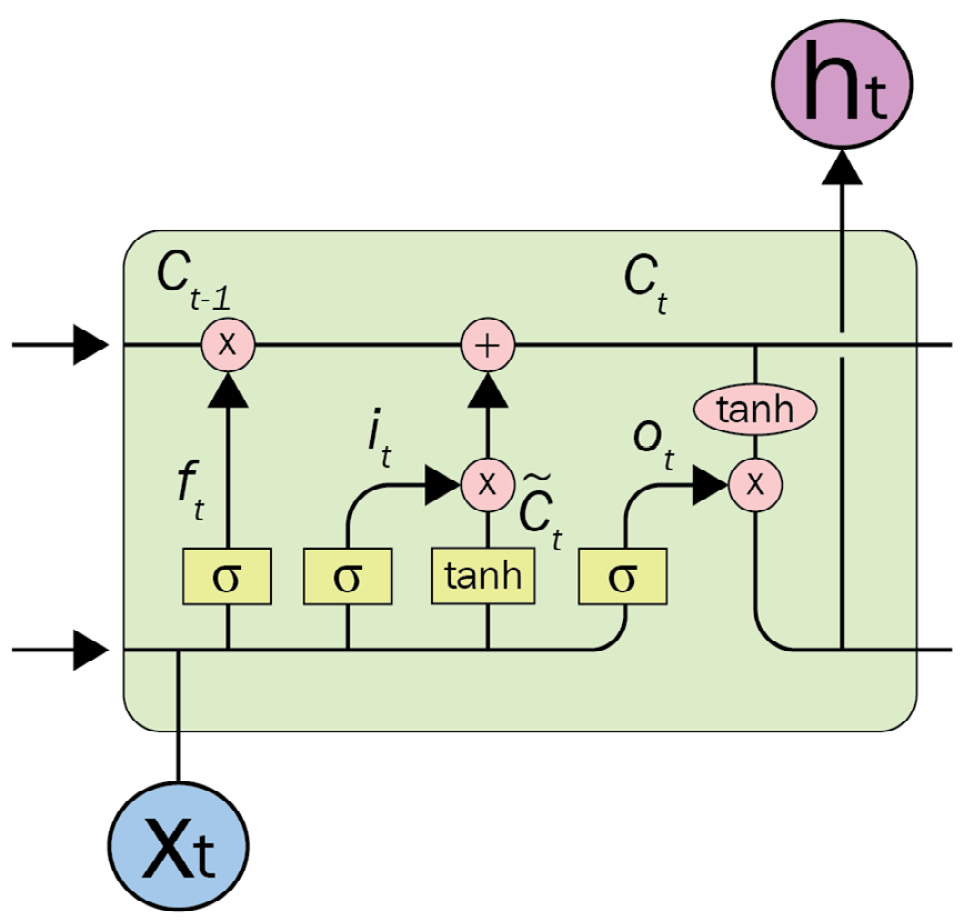Figure 1.6 – An LSTM unit

It is able to decide the following:

• What kind of information we will store in the cell state
• Which information will be forgotten or deleted

In the original RNN, in order to learn the state of I tokens, it recurrently processes the entire state of previous tokens between timestep0 and timestepi-1. Carrying entire information from earlier timesteps leads to vanishing gradient problems, which makes the model untrainable. The gate mechanism in LSTM allows the architecture to skip some unrelated tokens at a certain timestep or remember long-range states in order to learn the current token state.

A GRU is similar to an LSTM in many ways, the main difference being that a GRU does not use the cell state. Rather, the architecture is simplified by transferring the functionality of the cell state to the hidden state, and it only includes two gates: an update gate and a reset gate. The update gate determines how much information from the previous and current timesteps will be pushed forward. This feature helps the model keep relevant information from the past, which minimizes the risk of a vanishing gradient problem as well. The reset gate detects the irrelevant data and makes the model forget it.

### A gentle implementation of LSTM with Keras

We need to download the Stanford Sentiment Treebank (SST-2) sentiment dataset from the General Language Understanding Evaluation (GLUE) benchmark. We can do this by running the following code:

```\$ wget https://dl.fbaipublicfiles.com/glue/data/SST-2.zip
\$ unzip SST-2.zip```

Important note

SST-2: This is a fully labeled parse tree that allows for complete sentiment analysis in English. The corpus originally consists of about 12K single sentences extracted from movie reviews. It was parsed with the Stanford parser and includes over 200K unique phrases, each annotated by three human judges. For more information, see Socher et al., Parsing With Compositional Vector Grammars, EMNLP. 2013 (https://nlp.stanford.edu/sentiment).

```import tensorflow as tf
import pandas as pd
sentences=df.sentence
labels=df.label```

We need to set maximum sentence length, build vocabulary and dictionaries (`word2idx`, `idx2words`), and finally represent each sentence as a list of indexes rather than strings. We can do this by running the following code:

```max_sen_len=max([len(s.split()) for s in sentences])
list(set([w for s in sentences for w in s.split()]))
word2idx= {w:i for i,w in enumerate(words)}
max_words=max(word2idx.values())+1
idx2word= {i:w for i,w in enumerate(words)}
train=[list(map(lambda x:word2idx[x], s.split()))\
for s in sentences]```

Sequences that are shorter than `max_sen_len` (maximum sentence length) are padded with a `PAD` value until they are `max_sen_len` in length. On the other hand, longer sequences are truncated so that they fit `max_sen_len`. Here is the implementation:

```from keras import preprocessing
maxlen=max_sen_len)
Output: Train shape: (67349, 52)```

We are ready to design and train an LSTM model, as follows:

```from keras.layers import LSTM, Embedding, Dense
from keras.models import Sequential
model = Sequential()
model.compile(optimizer='rmsprop',loss='binary_crossentropy', metrics=['acc'])
history = model.fit(train_pad,labels, epochs=30, batch_size=32, validation_split=0.2)```

The model will be trained for 30 epochs. In order to plot what the LSTM model has learned so far, we can execute the following code:

```import matplotlib.pyplot as plt
def plot_graphs(history, string):
...
plot_graphs(history, 'acc')
plot_graphs(history, 'loss')```

The code produces the following plot, which shows us the training and validation performance of the LSTM-based text classification: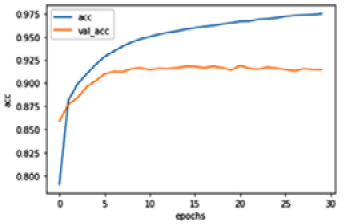Figure 1.7 – The classification performance of the LSTM network

As we mentioned before, the main problem of an RNN-based encoder-decoder model is that it produces a single fixed representation for a sequence. However, the attention mechanism allowed the RNN to focus on certain parts of the input tokens as it maps them to a certain part of the output tokens. This attention mechanism has been found to be useful and has become one of the underlying ideas of the Transformer architecture. We will discuss how the Transformer architecture takes advantage of attention in the next part and throughout the entire book.

## A brief overview of CNNs

CNNs, after their success in computer vision, were ported to NLP in terms of modeling sentences or tasks such as semantic text classification. A CNN is composed of convolution layers followed by a dense neural network in many practices. A convolution layer performs over the data in order to extract useful features. As with any DL model, a convolution layer plays the feature extraction role to automate feature extraction. This feature layer, in the case of NLP, is fed by an embedding layer that takes sentences as an input in a one-hot vectorized format. The one-hot vectors are generated by a `token-id` for each word forming a sentence. The left part of the following screenshot shows a one-hot representation of a sentence: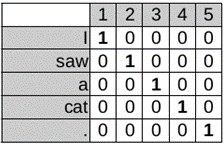Figure 1.8 – One-hot vectors

Each token, represented by a one-hot vector, is fed to the embedding layer. The embedding layer can be initialized by random values or by using pre-trained word vectors such as GloVe, Word2vec, or FastText. A sentence will then be transformed into a dense matrix in the shape of NxE (where N is the number of tokens in a sentence and E is the embedding size). The following screenshot illustrates how a 1D CNN processes that dense matrix: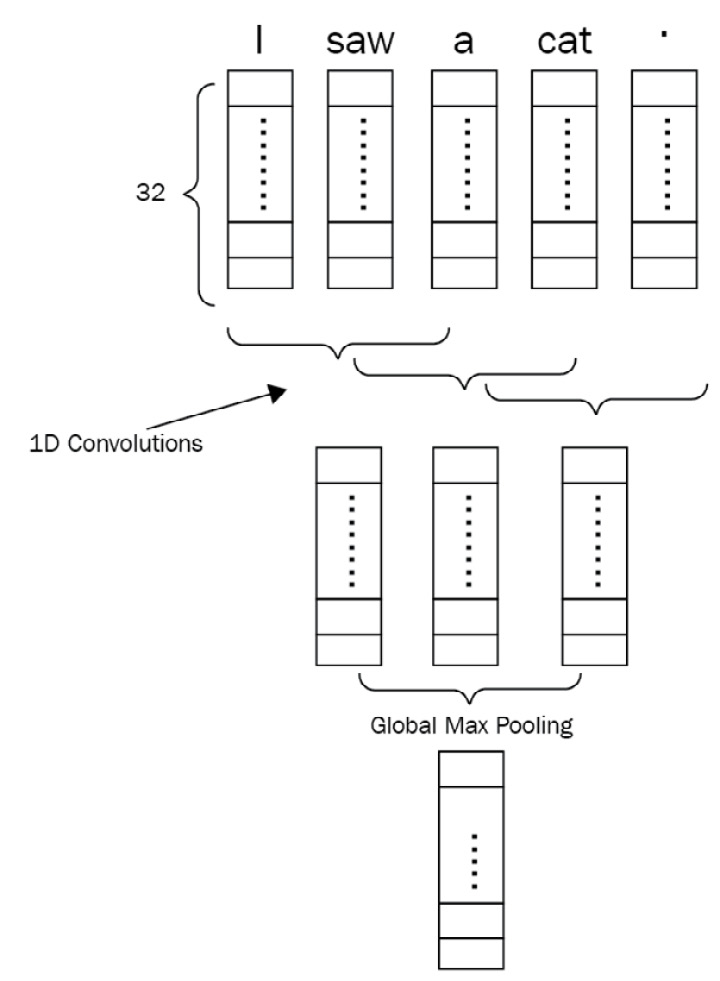Figure 1.9 – 1D CNN network for a sentence of five tokens

Convolution will take place on top of this operation with different layers and kernels. Hyperparameters for the convolution layer are the kernel size and the number of kernels. It is also good to note that 1D convolution is applied here and the reason for that is token embeddings cannot be seen as partial, and we want to apply kernels capable of seeing multiple tokens in a sequential order together. You can see it as something like an n-gram with a specified window. Using shallow TL combined with CNN models is also another good capability of such models. As shown in the following screenshot, we can also propagate the networks with a combination of many representations of tokens, as proposed in the 2014 study by Yoon Kim, Convolutional Neural Networks for Sentence Classification: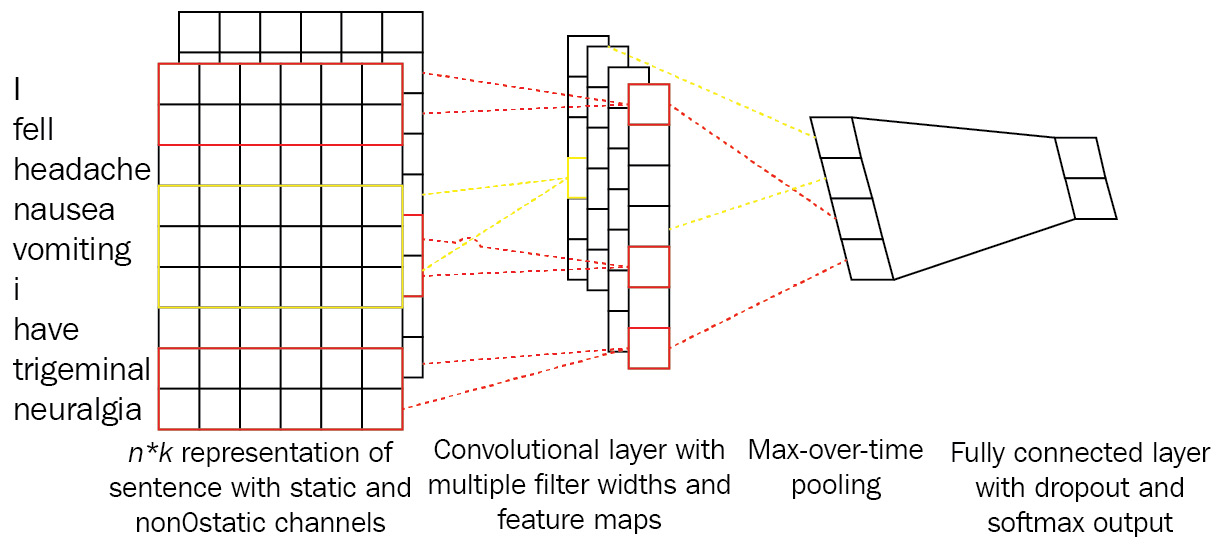Figure 1.10 – Combination of many representations in a CNN

For example, we can use three embedding layers instead of one and concatenate them for each token. Given this setup, a token such as fell will have a vector size of 3x128 if the embedding size is 128 for all three different embeddings. These embeddings can be initialized with pre-trained vectors from Word2vec, GloVe, and FastText. The convolution operation at each step will see N words with their respective three vectors (N is the convolution filter size). The type of convolution that is used here is a 1D convolution. The dimension here denotes possible movements when doing the operation. For example, a 2D convolution will move along two axes, while a 1D convolution just moves along one axis. The following screenshot shows the differences between them: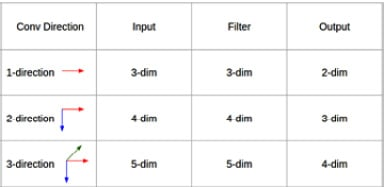Figure 1.11 – Convolutional directions

The following code snippet is a 1D CNN implementation processing the same data used in an LSTM pipeline. It includes a composition of `Conv1D` and `MaxPooling` layers, followed by `GlobalMaxPooling` layers. We can extend the pipeline by tweaking the parameters and adding more layers to optimize the model:

```from keras import layers
model = Sequential()
model.compile(loss='binary_crossentropy', metrics=['acc'])
history = model.fit(train_pad,labels, epochs=15, batch_size=32, validation_split=0.2)```

It turns out that the CNN model showed comparable performance with its LSTM counterpart. Although CNNs have become a standard in image processing, we have seen many successful applications of CNNs for NLP. While an LSTM model is trained to recognize patterns across time, a CNN model recognizes patterns across space.

# Overview of the Transformer architecture

Transformer models have received immense interest because of their effectiveness in an enormous range of NLP problems, from text classification to text generation. The attention mechanism is an important part of these models and plays a very crucial role. Before Transformer models, the attention mechanism was proposed as a helper for improving conventional DL models such as RNNs. To have a good understanding of Transformers and their impact on the NLP, we will first study the attention mechanism.

## Attention mechanism

One of the first variations of the attention mechanism was proposed by Bahdanau et al. (2015). This mechanism is based on the fact that RNN-based models such as GRUs or LSTMs have an information bottleneck on tasks such as Neural Machine Translation (NMT). These encoder-decoder-based models get the input in the form of a `token-id` and process it in a recurrent fashion (encoder). Afterward, the processed intermediate representation is fed into another recurrent unit (decoder) to extract the results. This avalanche-like information is like a rolling ball that consumes all the information, and rolling it out is hard for the decoder part because the decoder part does not see all the dependencies and only gets the intermediate representation (context vector) as an input.

To align this mechanism, Bahdanau proposed an attention mechanism to use weights on intermediate hidden values. These weights align the amount of attention a model must pay to input in each decoding step. Such wonderful guidance assists models in specific tasks such as NMT, which is a many-to-many task. A diagram of a typical attention mechanism is provided here: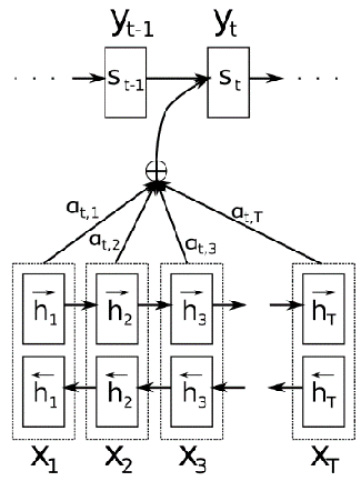Figure 1.12 – Attention mechanism

Different attention mechanisms have been proposed with different improvements. Additive, multiplicative, general, and dot-product attention appear within the family of these mechanisms. The latter, which is a modified version with a scaling parameter, is noted as scaled dot-product attention. This specific attention type is the foundation of Transformers models and is called a multi-head attention mechanism. Additive attention is also what was introduced earlier as a notable change in NMT tasks. You can see an overview of the different types of attention mechanisms here: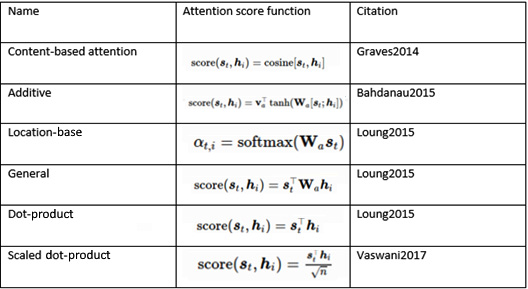Table 2 – Types of attention mechanisms (Image inspired from https://lilianweng.github.io/lil-log/2018/06/24/attention-attention.html)

Since attention mechanisms are not specific to NLP, they are also used in different use cases in various fields, from computer vision to speech recognition. The following screenshot shows a visualization of a multimodal approach trained for neural image captioning (K Xu et al., Show, attend and tell: Neural image caption generation with visual attention, 2015):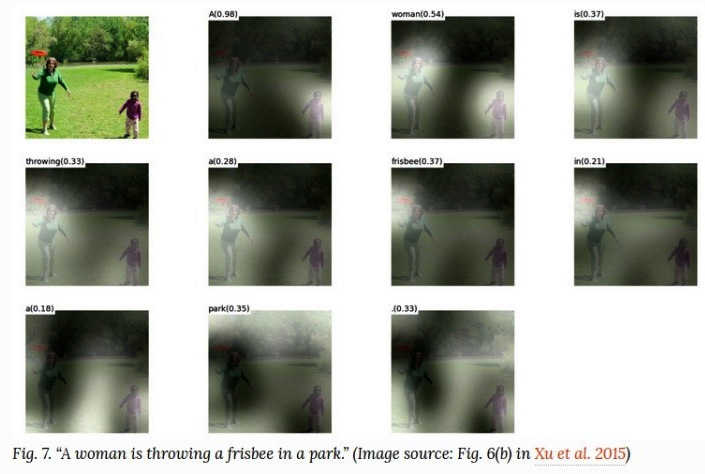Figure 1.13 – Attention mechanism in computer vision

The multi-head attention mechanism that is shown in the following diagram is an essential part of the Transformer architecture: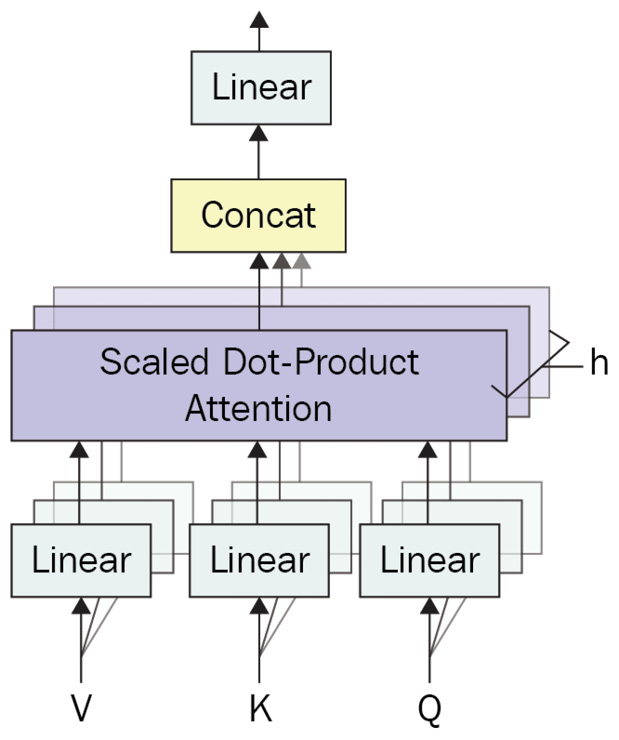Figure 1.14 – Multi-head attention mechanism

Next, let's understand multi-head attention mechanisms.

Before jumping into scaled dot-product attention mechanisms, it's better to get a good understanding of self-attention. Self-attention, as shown in Figure 1.15, is a basic form of a scaled self-attention mechanism. This mechanism uses an input matrix shown as X and produces an attention score between various items in X. We see X as a 3x4 matrix where 3 represents the number of tokens and 4 presents the embedding size. Q from Figure 1.15 is also known as the query, K is known as the key, and V is noted as the value. Three types of matrices shown as theta, phi, and g are multiplied by X before producing Q, K, and V. The multiplied result between query (Q) and key (K) yields an attention score matrix. This can also be seen as a database where we use the query and keys in order to find out how much various items are related in terms of numeric evaluation. Multiplication of the attention score and the V matrix produces the final result of this type of attention mechanism. The main reason for it being called self-attention is because of its unified input X; Q, K, and V are computed from X. You can see all this depicted in the following diagram: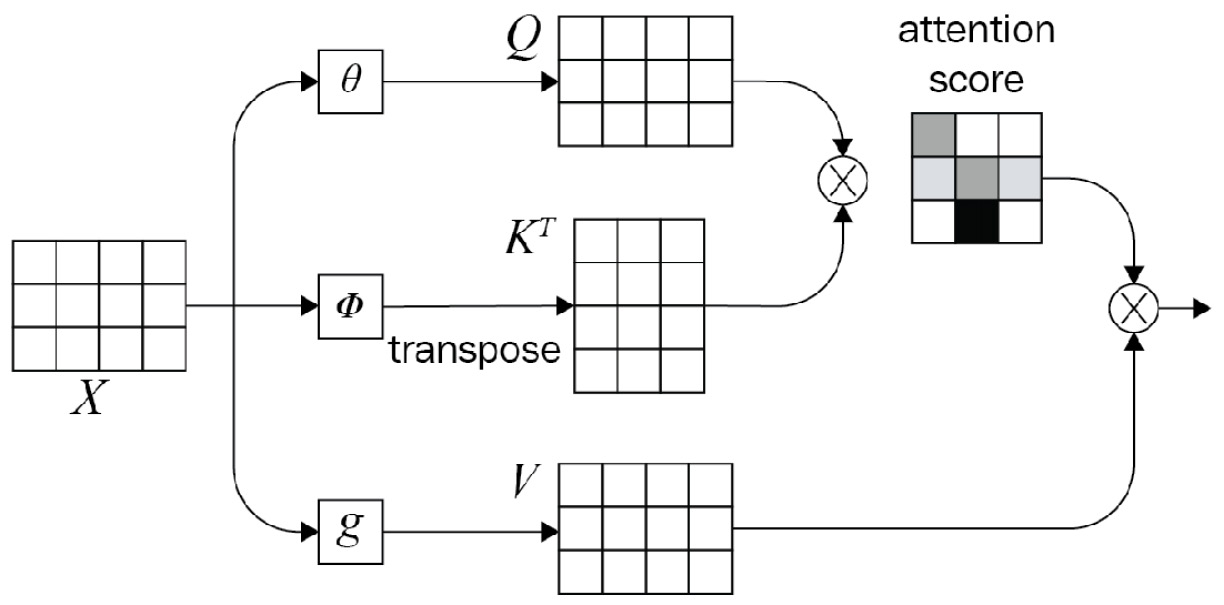Figure 1.15 – Mathematical representation for the attention mechanism (Image inspired from https://blogs.oracle.com/datascience/multi-head-self-attention-in-nlp)

A scaled dot-product attention mechanism is very similar to a self-attention (dot-product) mechanism except it uses a scaling factor. The multi-head part, on the other hand, ensures the model is capable of looking at various aspects of input at all levels. Transformer models attend to encoder annotations and the hidden values from past layers. The architecture of the Transformer model does not have a recurrent step-by-step flow; instead, it uses positional encoding in order to have information about the position of each token in the input sequence. The concatenated values of the embeddings (randomly initialized) and the fixed values of positional encoding are the input fed into the layers in the first encoder part and are propagated through the architecture, as illustrated in the following diagram: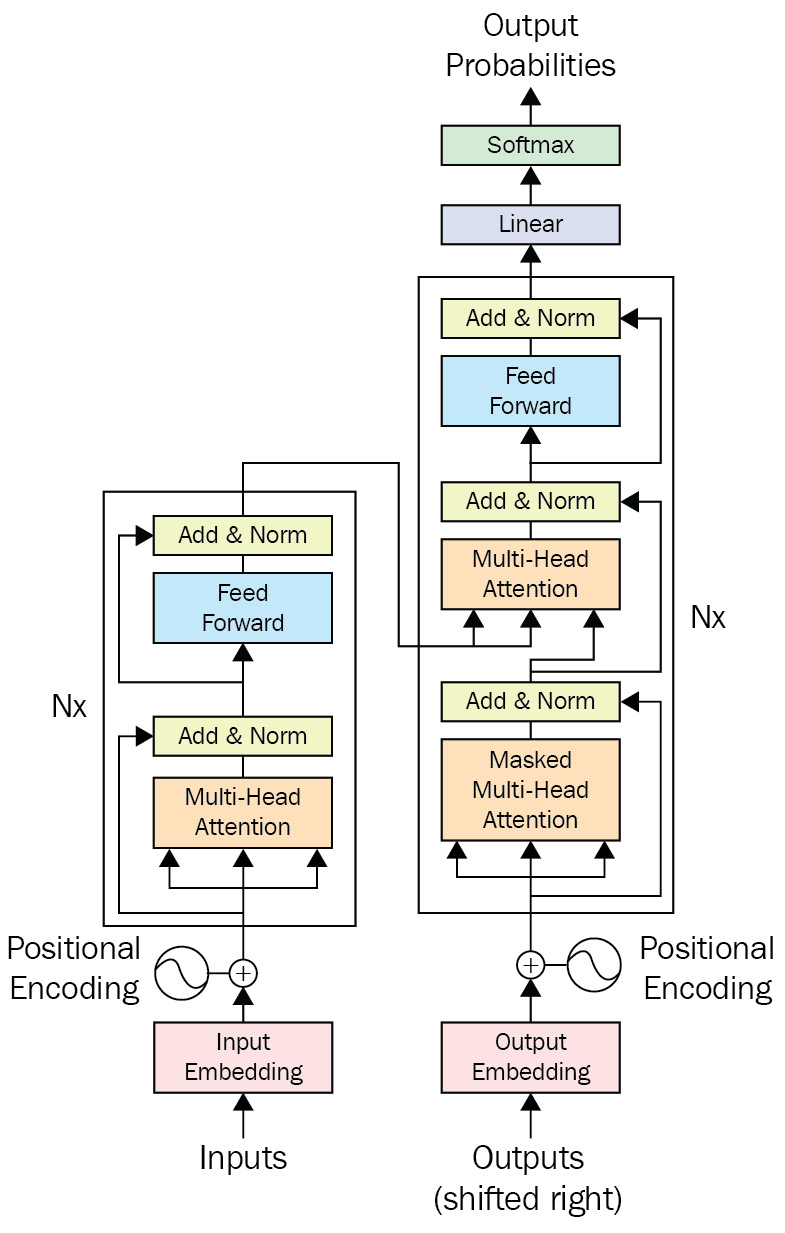Figure 1.16 – A Transformer

The positional information is obtained by evaluating sine and cosine waves at different frequencies. An example of positional encoding is visualized in the following screenshot: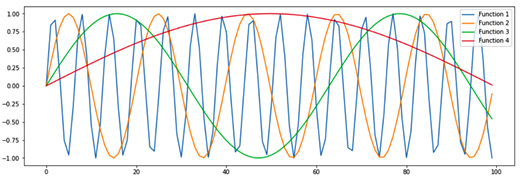Figure 1.17 – Positional encoding (Image inspired from http://jalammar.github.io/illustrated-Transformer/)

A good example of performance on the Transformer architecture and the scaled dot-product attention mechanism is given in the following popular screenshot: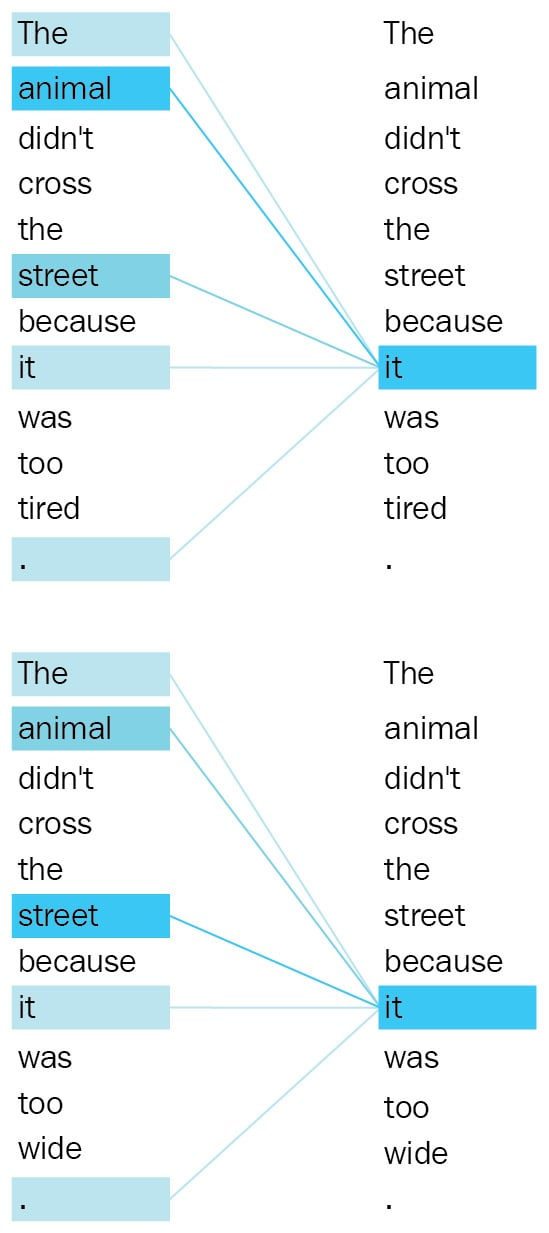Figure 1.18 – Attention mapping for Transformers (Image inspired from https://ai.googleblog.com/2017/08/Transformer-novel-neural-network.html)

The word it refers to different entities in different contexts, as is seen from the preceding screenshot. Another improvement made by using a Transformer architecture is in parallelism. Conventional sequential recurrent models such as LSTMs and GRUs do not have such capabilities because they process the input token by token. Feed-forward layers, on the other hand, speed up a bit more because single matrix multiplication is far faster than a recurrent unit. Stacks of multi-head attention layers gain a better understanding of complex sentences. A good visual example of a multi-head attention mechanism is shown in the following screenshot: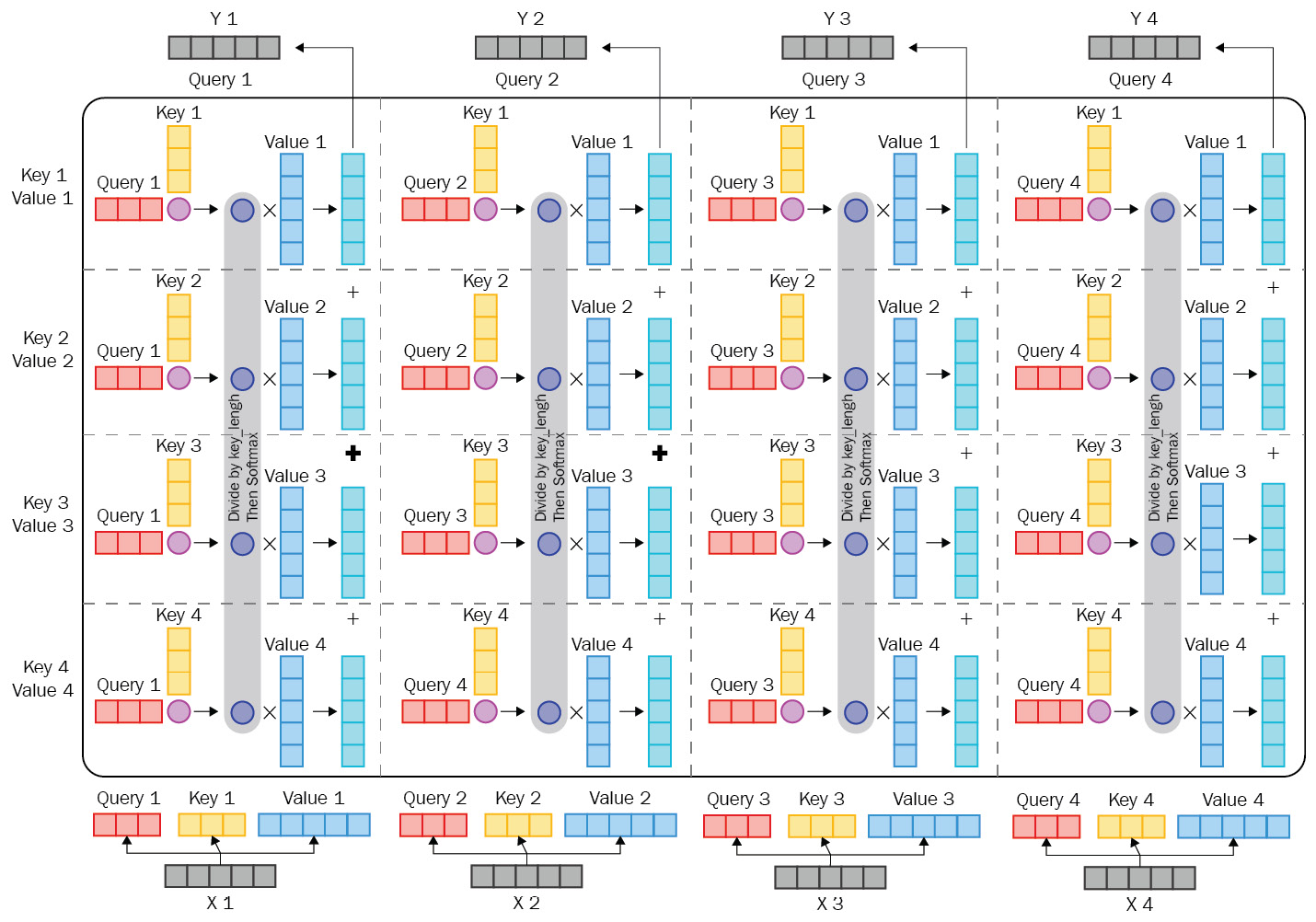Figure 1.19 – Multi-head attention mechanism (Image inspired from https://imgur.com/gallery/FBQqrxw)

On the decoder side of the attention mechanism, a very similar approach to the encoder is utilized with small modifications. A multi-head attention mechanism is the same, but the output of the encoder stack is also used. This encoding is given to each decoder stack in the second multi-head attention layer. This little modification introduces the output of the encoder stack while decoding. This modification lets the model be aware of the encoder output while decoding and at the same time help it during training to have a better gradient flow over various layers. The final softmax layer at the end of the decoder layer is used to provide outputs for various use cases such as NMT, for which the original Transformer architecture was introduced.

This architecture has two inputs, noted as inputs and outputs (shifted right). One is always present (the inputs) in both training and inference, while the other is just present in training and in inference, which is produced by the model. The reason we do not use model predictions in inference is to stop the model from going too wrong by itself. But what does it mean? Imagine a neural translation model trying to translate a sentence from English to French—at each step, it makes a prediction for a word, and it uses that predicted word to predict the next one. But if it goes wrong at some step, all the following predictions will be wrong too. To stop the model from going wrong like this, we provide the correct words as a shifted-right version.

A visual example of a Transformer model is given in the following diagram. It shows a Transformer model with two encoders and two decoder layers. The Add & Normalize layer from this diagram adds and normalizes the input it takes from the Feed Forward layer: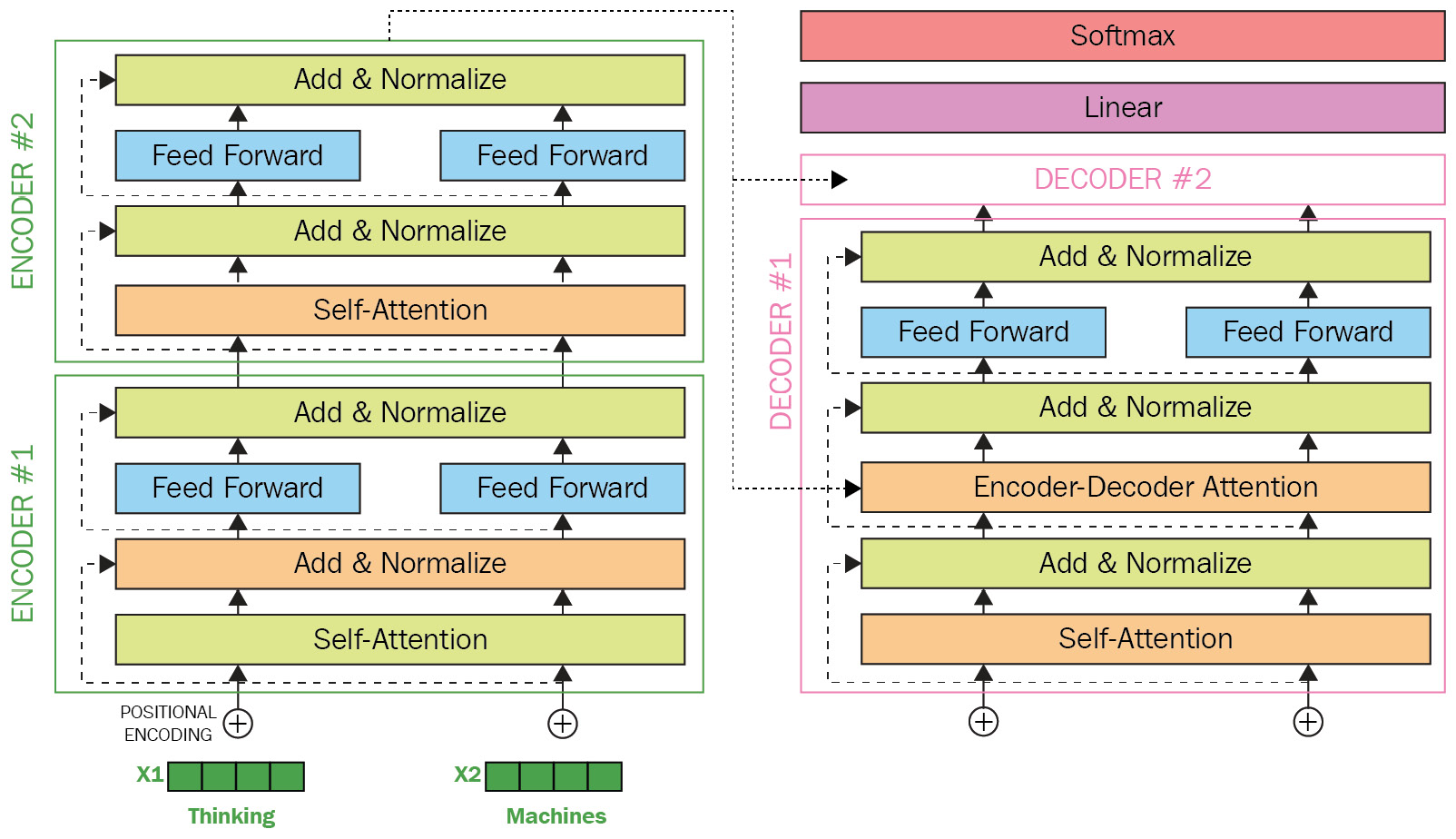Figure 1.20 – Transformer model (Image inspired from http://jalammar.github.io/illustrated-Transformer/)

Another major improvement that is used by a Transformer-based architecture is based on a simple universal text-compression scheme to prevent unseen tokens on the input side. This approach, which takes place by using different methods such as byte-pair encoding and sentence-piece encoding, improves a Transformer's performance in dealing with unseen tokens. It also guides the model when the model encounters morphologically close tokens. Such tokens were unseen in the past and are rarely used in the training, and yet, an inference might be seen. In some cases, chunks of it are seen in training; the latter happens in the case of morphologically rich languages such as Turkish, German, Czech, and Latvian. For example, a model might see the word training but not trainings. In such cases, it can tokenize trainings as training+s. These two are commonly seen when we look at them as two parts.

Transformer-based models have quite common characteristics—for example, they are all based on this original architecture with differences in which steps they use and don't use. In some cases, minor differences are made—for example, improvements to the multi-head attention mechanism taking place.

# Using TL with Transformers

TL is a field of Artificial Intelligence (AI) and ML that aims to make models reusable for different tasks—for example, a model trained on a given task such as A is reusable (fine-tuning) on a different task such as B. In an NLP field, this is achievable by using Transformer-like architectures that can capture the understanding of language itself by language modeling. Such models are called language models—they provide a model for the language they have been trained on. TL is not a new technique, and it has been used in various fields such as computer vision. ResNet, Inception, VGG, and EfficientNet are examples of such models that can be used as pre-trained models able to be fine-tuned on different computer-vision tasks.

Shallow TL using models such as Word2vec, GloVe, and Doc2vec is also possible in NLP. It is called shallow because there is no model behind this kind of TL and instead, the pre-trained vectors for words/tokens are utilized. You can use these token- or document-embedding models followed by a classifier or use them combined with other models such as RNNs instead of using random embeddings.

TL in NLP using Transformer models is also possible because these models can learn a language itself without any labeled data. Language modeling is a task used to train transferable weights for various problems. Masked language modeling is one of the methods used to learn a language itself. As with Word2vec's window-based model for predicting center tokens, in masked language modeling, a similar approach takes place, with key differences. Given a probability, each word is masked and replaced with a special token such as [MASK]. The language model (a Transformer-based model, in our case) must predict the masked words. Instead of using a window, unlike with Word2vec, a whole sentence is given, and the output of the model must be the same sentence with masked words filled.

One of the first models that used the Transformer architecture for language modeling is BERT, which is based on the encoder part of the Transformer architecture. Masked language modeling is accomplished by BERT by using the same method described before and after training a language model. BERT is a transferable language model for different NLP tasks such as token classification, sequence classification, or even question answering.

Each of these tasks is a fine-tuning task for BERT once a language model is trained. BERT is best known for its key characteristics on the base Transformer encoder model, and by altering these characteristics, different versions of it—small, tiny, base, large, and extra-large—are proposed. Contextual embedding enables a model to have the correct meaning of each word based on the context in which it is given—for example, the word Cold can have different meanings in two different sentences: Cold-hearted killer and Cold weather. The number of layers at the encoder part, the input dimension, the output embedding dimension, and the number of multi-head attention mechanisms are these key characteristics, as illustrated in the following screenshot: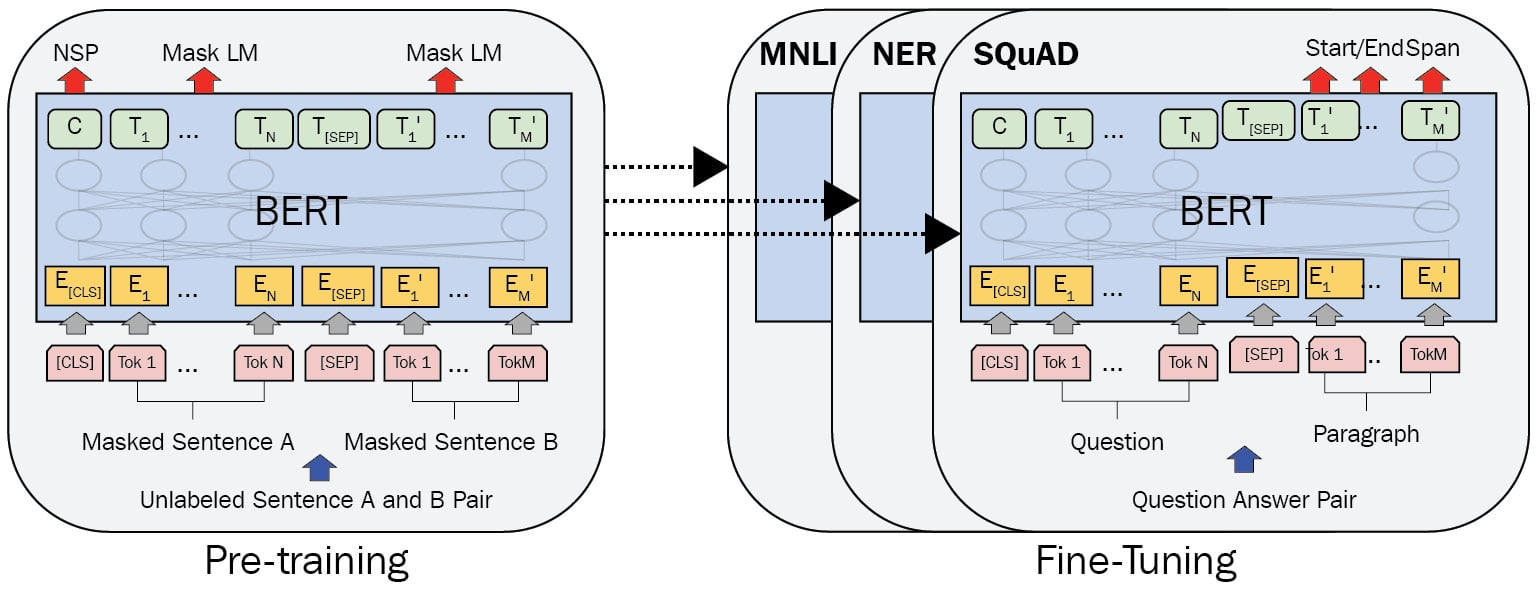Figure 1.21 – Pre-training and fine-tuning procedures for BERT (Image inspired from J. Devlin et al., Bert: Pre-training of deep bidirectional Transformers for language understanding, 2018)

As you can see in Figure 1.21, the pre-training phase also consists of another objective known as next-sentence prediction. As we know, each document is composed of sentences followed by each other, and another important part of training for a model to grasp the language is to understand the relations of sentences to each other—in other words, whether they are related or not. To achieve these tasks, BERT introduced special tokens such as [CLS] and [SEP]. A [CLS] token is an initially meaningless token used as a start token for all tasks, and it contains all information about the sentence. In sequence-classification tasks such as NSP, a classifier on top of the output of this token (output position of 0) is used. It is also useful in evaluating the sense of a sentence or capturing its semantics—for example, when using a Siamese BERT model, comparing these two [CLS] tokens for different sentences by a metric such as cosine-similarity is very helpful. On the other hand, [SEP] is used to distinguish between two sentences, and it is only used to separate two sentences. After pre-training, if someone aims to fine-tune BERT on a sequence-classification task such as sentiment analysis, which is a sequence-classification task, they will use a classifier on top of the output embedding of [CLS]. It is also notable that all TL models can be frozen during fine-tuning or freed; frozen means seeing all weights and biases inside the model as constants and stopping training on them. In the example of sentiment analysis, just the classifier will be trained, not the model if it is frozen.

# Summary

With this, we now come to the end of the chapter. You should now have an understanding of the evolution of NLP methods and approaches, from BoW to Transformers. We looked at how to implement BoW-, RNN-, and CNN-based approaches and understood what Word2vec is and how it helps improve the conventional DL-based methods using shallow TL. We also looked into the foundation of the Transformer architecture, with BERT as an example. By the end of the chapter, we had learned about TL and how it is utilized by BERT.

At this point, we have learned basic information that is necessary to continue to the next chapters. We understood the main idea behind Transformer-based architectures and how TL can be applied using this architecture.

In the next section, we will see how it is possible to run a simple Transformer example from scratch. The related information about the installation steps will be given, and working with datasets and benchmarks is also investigated in detail.

# References

• Mikolov, T., Chen, K., Corrado, G. & Dean, J. (2013). Efficient estimation of word representations in vector space. arXiv preprint arXiv:1301.3781.
• Bahdanau, D., Cho, K. & Bengio, Y. (2014). Neural machine translation by jointly learning to align and translate. arXiv preprint arXiv:1409.0473.
• Pennington, J., Socher, R. & Manning, C. D. (2014, October). GloVe: Global vectors for word representation. In Proceedings of the 2014 conference on empirical methods in natural language processing (EMNLP) (pp. 1532-1543).
• Hochreiter, S. & Schmidhuber, J. (1997). Long short-term memory. Neural computation, 9(8), 1735-1780.
• Bengio, Y., Simard, P, & Frasconi, P. (1994). Learning long-term dependencies with gradient descent is difficult. IEEE transactions on neural networks, 5(2), 157-166.
• Cho, K., Van Merriënboer, B., Gulcehre, C., Bahdanau, D., Bougares, F., Schwenk, H. & Bengio, Y. (2014). Learning phrase representations using RNN encoder-decoder for statistical machine translation. arXiv preprint arXiv:1406.1078.
• Kim, Y. (2014). Convolutional neural networks for sentence classification. CoRR abs/1408.5882 (2014). arXiv preprint arXiv:1408.5882.
• Vaswani, A., Shazeer, N., Parmar, N., Uszkoreit, J., Jones, L., Gomez, A. N. & Polosukhin, I. (2017). Attention is all you need. arXiv preprint arXiv:1706.03762.
• Devlin, J., Chang, M. W., Lee, K. & Toutanova, K. (2018). Bert: Pre-training of deep bidirectional Transformers for language understanding. arXiv preprint arXiv:1810.04805.

• ##### Savaş Yıldırım

Savaş Yıldırım graduated from the Istanbul Technical University Department of Computer Engineering and holds a Ph.D. degree in Natural Language Processing (NLP). Currently, he is an associate professor at the Istanbul Bilgi University, Turkey, and is a visiting researcher at the Ryerson University, Canada. He is a proactive lecturer and researcher with more than 20 years of experience teaching courses on machine learning, deep learning, and NLP. He has significantly contributed to the Turkish NLP community by developing a lot of open source software and resources. He also provides comprehensive consultancy to AI companies on their R&D projects. In his spare time, he writes and directs short films, and enjoys practicing yoga.

Browse publications by this author
• ##### Meysam Asgari-Chenaghlu

Meysam Asgari-Chenaghlu is an AI manager at Carbon Consulting and is also a Ph.D. candidate at the University of Tabriz. He has been a consultant for Turkey's leading telecommunication and banking companies. He has also worked on various projects, including natural language understanding and semantic search.

Browse publications by this author

### Latest Reviews

(3 reviews total)